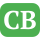CppBuzz.com

# Union in C Programming

The union is a user defined data type in C programming. It can have n numbers of members but only one member can be accessed at one time. The union was invented to prevent memory fragmentation. The union data type prevents fragmentation by creating a standard size for certain data. The members of union can be accessed with . (dot) or -> operators.

## Example on union

```#include<stdio.h>
union myunion
{
float A;
int B;
};

int main()
{
union myunion numbers;
numbers.A = 7.5;
numbers.B = 77;

printf("\n Size of : %d", sizeof(numbers));

return 0;
}
```

## Typedef with union

```#include<stdio.h>

typedef union myunion
{
float A;
int B;
}MYUNION;

int main()
{
MYUNION numbers;
numbers.A = 7.5;
numbers.B = 77;

printf("\n Size of : %d", sizeof(numbers));

return 0;
}
```

## Accessing Members of union

```#include<stdio.h>

typedef union myunion
{
float A;
int B;
}MYUNION;

int main()
{
MYUNION numbers;
numbers.A = 7.5;
numbers.B = 77;

printf("\n A is : %f", numbers.A);
printf("\n B is : %d", numbers.B);

return 0;
}
```

## One member should be accessed at one time in union

```#include<stdio.h>

typedef union myunion
{
float A;
int B;
}MYUNION;

int main()
{
MYUNION numbers;
numbers.A = 7.5;

printf("\n A is : %f", numbers.A);

numbers.B = 77;
printf("\n B is : %d", numbers.B);

return 0;
}
```